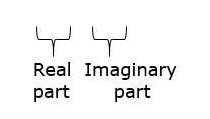# Simplify each of the following and express it in the form (a + ib) :

Question:

Simplify each of the following and express it in the form (a + ib) :

$(5-2 i)^{2}$

Solution:

Given: $(5-2 i)^{2}$

We know that

$(a-b)^{2}=a^{2}+b^{2}-2 a b \ldots(i)$

So, on replacing a by 5 and b by 2i in eq. (i), we get

$(5)^{2}+(2 i)^{2}-2(5)(2 i)$

$=25+4 \mathrm{i}^{2}-20 \mathrm{i}$

$=25-4-20 \mathrm{i}\left[\because \mathrm{i}^{2}=-1\right]$

$=21-20 \mathrm{i}$